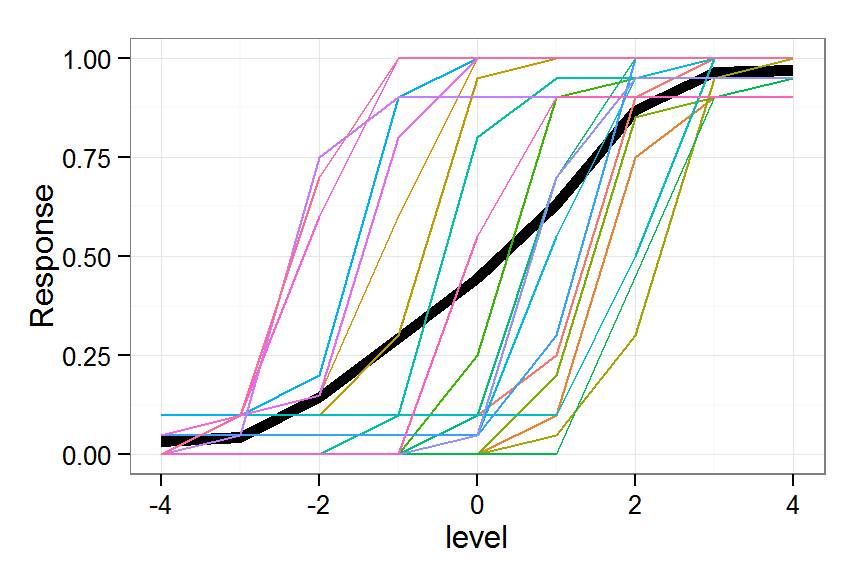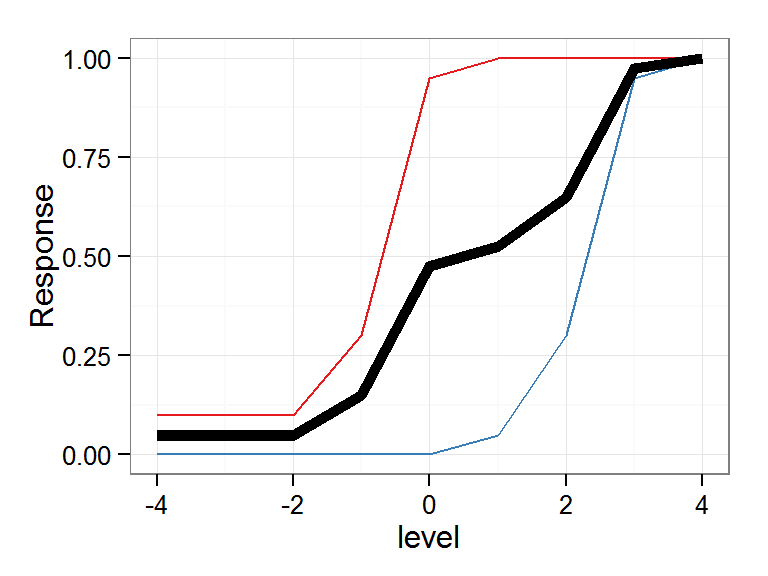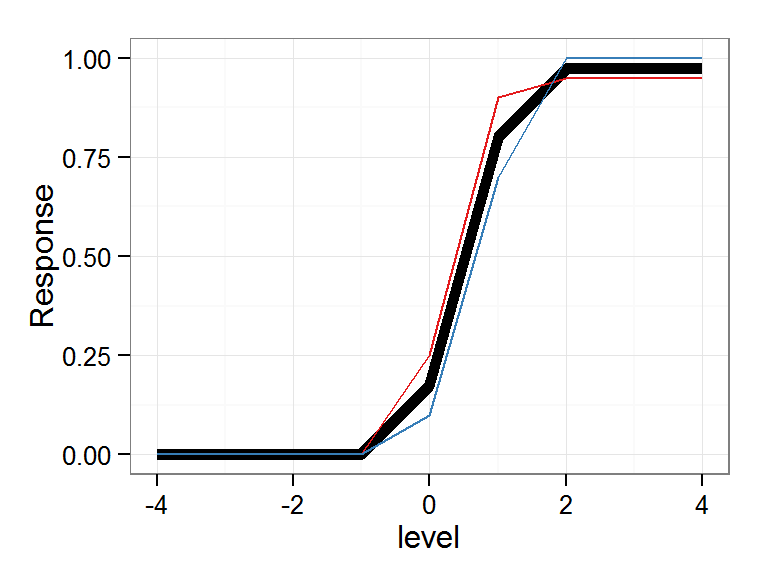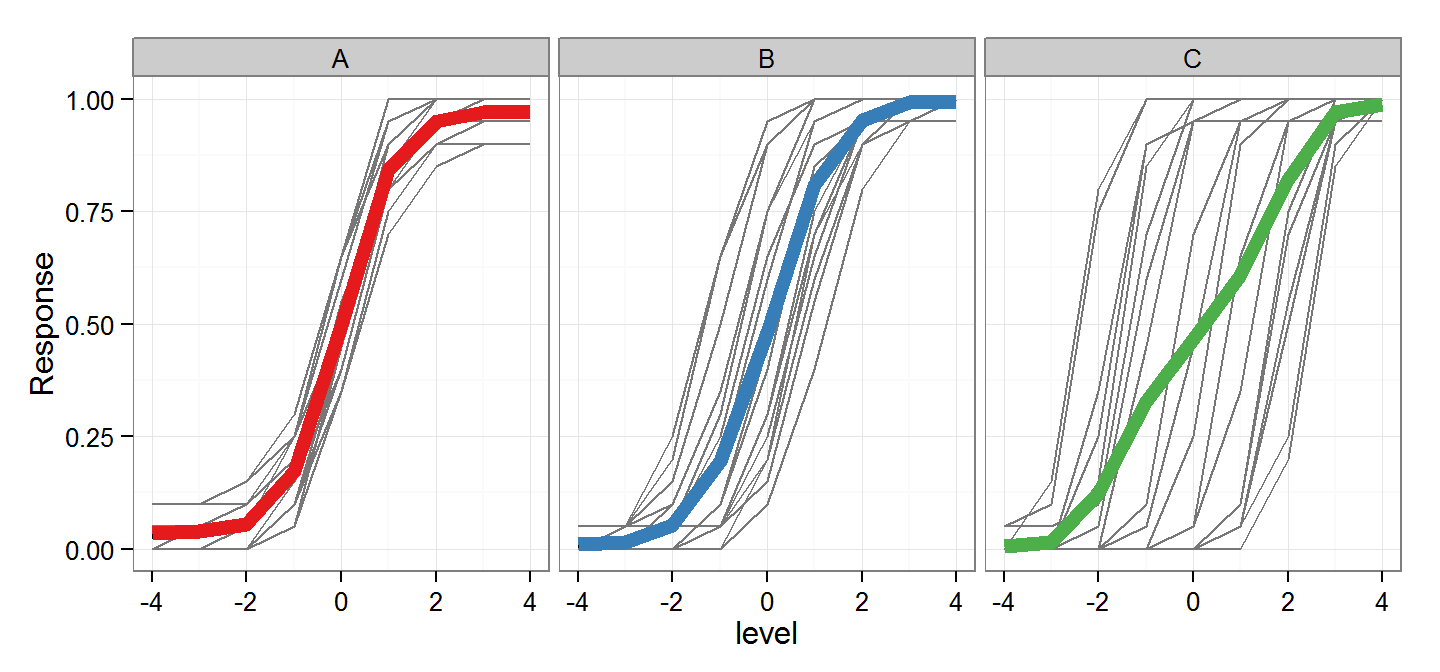Take a look at this plot:This plot displays the average binomial (0 or 1) response for many different participants who respond to a stimulus whose values span across the X axis. Each participant is a different color.
The group mean response is the thick black line. It is the arithmetic mean of all the responses, and yet it doesn’t come close to representing any of the individual listeners.
This is a simple consequence of averaging. It’s easier to see in this plot:At level 0, the red line is near the extreme top, while the blue line is near the extreme bottom. the average is (unsurprisingly) right in the middle.
In another post, we looked at context effects, which are essentially differences among intercepts, which you can see as horizontal differences between response functions.

### Here’s the problem:

In many experiments, we want to estimate the slope of these functions. The slopes of both the red and blue lines are rather steep, but the slope of their average is less steep. This is inevitable if they are offset along the X axis.
The X-axis offset can be considered a bias, which is separate from the slope. In regular binomial GLM, the bias is measured as the intercept.

## Intercept. Now we’re getting somewhere. If we treat red and blue as members of the same group and believe that the group has one underlying intercept, then we are forced to accept the averaged thick black line as the best estimate of the group effect.
However, people are… people. They vary in many ways, and their behavior in perceptual experiments can reflect a distribution of biases (as well as slopes). that introduce such divergence of response curves.
By treating the intercept as a random effect of Subject, we acknowledge that, while there may be some variance in the impact of the factor that we are measuring, there is also variance attributable to the particular people that we sampled, which is not useful for estimating the interesting effect.
## You’re talking about mixed-effects modeling. Yes.
Imagine that the extreme divergence of the red and blue curves were shrunk toward the centerNow the group average line better reflects the component curves

### Who cares?

This makes a difference when estimating slopes of groups who vary widely in their intercepts (as in the big plot at the top of this page).
Consider these three groups:It’s easy to see that the group averages of these three conditions is roughly equal, but the individual curves are noticably different.

In particular, we can see that the individual curves in panel C are steeper than those in panels A and B.
Why isn’t this reflected in the group average (thick line)?
For the same reason we discussed above. The difference in intercept shallows out the average slope.
## Modeling
First, think about the conventional approach:

# basic GLM
mod.glm <- glm(Response ~ level*condition, data=data.all, family="binomial")
coefs.glm <- coefficients(summary(mod.glm))
coefs.glm
##                  Estimate Std. Error z value   Pr(>|z|)
## (Intercept)      -0.11802    0.05660 -2.0852  3.705e-02
## level             1.26797    0.04126 30.7280 2.408e-207
## conditionB        0.14744    0.08045  1.8326  6.687e-02
## conditionC       -0.46185    0.07805 -5.9173  3.273e-09
## level:conditionB  0.03244    0.05934  0.5466  5.846e-01
## level:conditionC -0.24403    0.05223 -4.6719  2.984e-06

See how the coefficient summary tells us that the slope for Condition C is lower?

Actually, the summary doesn’t tell us that directly - we have to do a little mental math.

Specifically, the coefficient for Condition C slope is the Estimate for level plus the Estimate for level:conditionC. That second term (the interaction) tells you how different the slope for Condition C is relative to the default condition. It is negative, meaning that the effect is weaker (shallower slope).

We can plainly see that this output does not capture what we see to be true in the data: that the slopes in Condition C tend to be steeper than those in the other conditions.

## Now what do we do? Here’s a GLMER with random intercept for each subject in each condition

mod.glmer <- glmer(Response ~ level*condition +
(1|Subject) + (1|condition:Subject),
data=data.all, family="binomial")
coefs.glmer <- coefficients(summary(mod.glmer))
coefs.glmer
##                  Estimate Std. Error z value   Pr(>|z|)
## (Intercept)       -0.1237    0.50869 -0.2431  8.079e-01
## level              1.3297    0.04400 30.2189 1.338e-200
## conditionB         0.1589    0.69806  0.2277  8.199e-01
## conditionC        -1.2147    0.70174 -1.7310  8.345e-02
## level:conditionB   0.2281    0.07022  3.2477  1.164e-03
## level:conditionC   1.0806    0.10878  9.9343  2.953e-23

Notice how the level:conditionC term is now in the correct (positive) direction, indicating that the slope for Condition C is steeper than that observed in the default condition.

## What have we done?

We have refused to let a little thing like intercept variance get in the way of a good slope estimation.

This is an R Markdown document. Markdown is a simple formatting syntax for authoring HTML, PDF, and MS Word documents. For more details on using R Markdown see http://rmarkdown.rstudio.com.